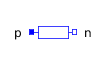Absolute Rotation - MapleSim Help

Absolute Rotation

Measures the absolute rotational motion of a frame with respect to groundDescription An absolute rotation sensor measures the rotation matrix, angular velocity, and angular acceleration of a frame with respect to ground. The sensor generates three vector outputs (a length 9 vector (column-major order) and two 3-vectors) containing these measurements, expressed in the frame specified by the Resolved Frame parameter. (Applies to the angular velocity and acceleration only. This parameter is ignored for the rotation matrix output.)Connections

 Name Description ${\mathrm{frame}}_{b}$ Frame to measure $\mathrm{RMOutputR}$ Real signal of dimension 9 (column-major order) that represents the rotation matrix between the inertial and frame_b. $\mathrm{RMOutputW}$ Real signal of dimension 3 that specifies the angular velocity of frame_b with respect to the Inertial frame.  The measured quantity is expressed in the frame specified by the Resolved Frame parameter. $\mathrm{RMOutputAA}$ Real signal of dimension 3 that specifies the angular acceleration of frame_b with respect to the Inertial frame.  The measured quantity is expressed in the frame specified by the Resolved Frame parameter.Parameters

 Symbol Default Units Description Modelica ID Resolved Frame Inertial Frame in which the output signals are expressed. FrameSee Also